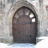# Calculations with null values

357
2
06-25-2018 04:46 AMOccasional Contributor

I have a feature class with four fields (A1, A2, B1, B2) of type Double - each field has values as well as nulls. I want to be able to populate a second set of fields (A1A2, B1B2) with the sum of the values e.g. A1A2 = A1+A2, B1B2=B1+B2.. A simple addition using Field Calculator fails where A1 and/or A2 are nulls (which I expected). I can  copy values to another field using a python expression to deal with nulls -

Prelogic Script Code

def updateValue(value):
if value == None:
return '0'
else: return value

and expression

A1A2 = (updateValue(!A1!))

(this copies A1 to A1A2 field and changes the nulls to 0 in the process.

If I change the expression to try and add two values ( i.e.  A1A2 = (updateValue(!A1!)) + (updateValue(!A2!)) ), this works when both A1 and A2 are not nulls, but falls over if either or both fields are null (gives a value in A1A2 of null).

Can someone point me towards a calculator expression (or other method) of achieving this result. I want to have the expression run within a model, with the intent to have a process that I am presently doing part manually  setup as a model so that a basic user (one of my managers) can run herself when requiredwithout bothering me.all the time. I should be able to  set it up so that I have new fields e.g. tmpA1 and tmpA2 which are populated with the A1 and A2 values with nulls changed to 0, and then perform the addition (and will implement this if required), but to me this is a bit of a kludge and would like to have a more elegant solution.  I want to maintain the original fields with their null values as these are meaningful ( null is not 0). I feel a solution should be possible within field calculator but understand that this may or may not be possible, in which case I am willing would try something else.

I have some modelbuilder skills (more like - will this work yes/no if no then try something else) without necessarily a deep understanding of what I am doing, and no real python knowledge or coding background.

2 RepliesbyMVP Legendary Contributor

You never check to see if something is equal to None, you check to see if something IS None.

So if both are not None, then do something with them, like add them

``````def updateValue(v0, v1):
if (v0 is not None) and (v1 is not None):
return v0 + v1
else:
return 0‍‍‍‍‍``````byEsri Esteemed Contributor

You could try something like this I suppose:

``````def main():

a1 = 2.5
a2 = None
b1 = None
b2 = 1.23

print(SumValues([a1, a2]))
print(SumValues([b1, b2]))
print(SumValues([a1, b2]))
print(SumValues([a2, b1]))

def SumValues(lst_val):
lst_val = [a for a in lst_val if a != None]
try:
return sum(lst_val)
except:
return 0

if __name__ == '__main__':
main()‍‍‍‍‍‍‍‍‍‍‍‍‍‍‍‍‍‍‍‍‍``````

This will yield:

``````2.5
1.23
3.73
0‍‍‍‍``````